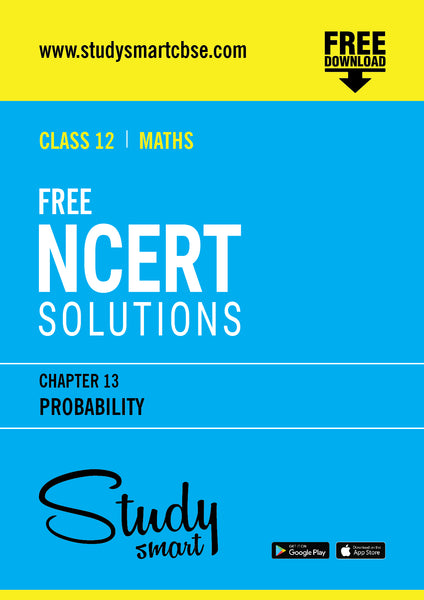# 13. Probability

Your are buying an E-Book (PDF)
This will be sent as a link to your registered email id

#### Description:

Conditional probability, multiplication theorem on probability, independent events, total probability, Bayes’ theorem, Random variable and its probability distribution, mean and variance of random variable. Repeated independent (Bernoulli) trials and Binomial distribution.